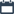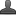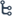## Tuesday, October 27, 2020

•October 27, 2020
••, ,
•No comments

## Important questions for 10th class Maths chapter-6 Progression,Mathematics questionnaire-x class

6.PROGRESSIONS

Hi friends and my dear students! In this post, I have covered Important questions for 10th class maths chapter-6.Progression,Chapter wise previous year Questions. After Reading  Please do share it with your friends. Learn maths for All classes her

PRIORITY-I

1 MARK QUESTIONS:

1. Write first three terms of the AP when a=4 and d=-3?

2. Find d of the AP ¼,-1/4,-3/4,-5/4………?

3. Find d of the AP  √2, √ 8,√18, √32….?

4. Find the 10th term of the AP 5, 1, -3, - 7,…………..?

5. Which term of the AP 21, 18, 15, ……………………… is -81 ?

6. Find the sum of first 100 natural numbers?

7. Write the GP, if a=3, and r=2 ?

8. Check weather 301 is a term of the list of number 5,11,27,23,……

9. Find the sum of -1,1/4,3/2..(10 terms)

10. Which term of the G.P; 2, 2√2,4…..is 128?

11. In an A.P the sum of n terms is always not equal to zero say your opinion on this statement?

12. If x,x+2,x+3 are consecutive terms of a G.P. then find x.

13. Find the common ratio of the GP 25,-5, 1, -1/5, …………………?

14. Find x so that x,x+2, x+6 are consecutive terms of GP ?

15. Find the 10th term of the GP 5,25,125….?

2 MARK QUESTIONS:

13. Determine the AP whose 3rd term is 5 and the 7th term is 9. ?

14. How many two digits numbers are divisible by 3?

15. Find the 11th term from the last of the A.P series 10,7,4……62

16. How many multiples of 4 lie between 10 and 250?

17. Find the sum of the first 40 positive integers divisible by 6?

18. If 3rd and 9th terms of a n A.P are 4 and -8 respectively which term of the A.P is zero

19. The first term and the last terms of an A.P are 17 and 350 respectively if the common difference is 9 how many terms are there and what is their sum?

20. Which term of AP 3, 8, 13, 18, …………………..78?

21. Find the 31st term of an AP whose 11th term is 38 and 16th term is 73. ?

22. Find the 20th term from the end of the AP 3,8, 13,……………….., 253?

23. Subbarao started work in 1995 at annual salary of Rs.5000 and received an increment of Rs.200 each year. In which year did his income reach Rs.7000?

24. If the sum of the first 14 terms of an AP is 1050 and its first term is 10. Find the 20th term. ?

25. How many terms of the AP 24,21,18,……..must be taken so that their sum is 78 .?

26. Find the sum of first 24 terms of the list of numbers whose nth term is given by an = 3+2n ?

27. Find the 20th term and nth term of the GP5/2,5/4 ,5/8  ………?

28. In a GP the 3rd term is 24 and 6th term is 192. Find the 10th term ?

29. Deduce sum to n terms in an A.P. by Gauss?

Also Check

Important questions for 10th class maths chapter-5 Quadratic-equations

## Important questions for 10th class maths chapter-1 Real numbersImportant questions for 10th class maths chapter-4 LINEAR EQUATIONSImportant questions for 10th class maths chapter-3 polynomial

4 MARKS QUESTIONS

1. A sum of Rs.1000 is invested at 8% simple interest per year. Calculate the interest at the end of each year. Do these interests form an AP? If so, find the interest at the end of 30 years?

2. In a flower bed, there are 23 rose plants in the first row, 21 in the second row, 19 in the third row and so on. There are 5 rose plants in the last row. How many rows are there in the flower bed.?

3. A manufacture of TV sets produced 600sets in the third year and 700 sets in the seventh year. Assuming that the production increases uniformly by a fixed number every year, find

(i) the production in the 1st year? (ii) the production in the 10th year? (iii)the total production in 7 years?

4. The sum of the 4th and 8th terms of an AP is 24 and the sum of the 6th and 10th terms is 44. Find the first three terms of the AP.

5. A sum of 700/- is to be used to give seven cash prizes to students of a school for their overall academic performance if each prize is 20/- less than its preceding prize find the value of each of the prizes.

6. The 4th term of a GP is 2/3 and the 7th term is 16/81. Find the GP?

7. If the geometric progressions 162,54,18…. And 2/87,2/27,2/9…………….have their nth term find the value of ‘n’

PRIORITY-II

2 MARK QUESTIONS:

1. Which term of the GP 2,8,32,---is 512?

2. Is 84 is a term of the sequence 3,7,11----?

3. In 56,63,70,---497 series how many terms are there?

4 MARKS QUESTIONS:

1. A Contractor constriction job specifies a penalty for delay of completion beyond a certain date as follows Rs. 200 for the first day. The penalty for each succeeding day being Rs 50 more than the preceding day. How much money does the contractor pay as penalty if he has delayed the work by 30 days

2. A spiral is made up of successive semicircles, with centers alternately at A and B, starting with centre at A, of radius 0.5 cm, 1.0 cm, 1.5 cm, 2.0 cm, . . . What is the total length of such a spiral made up of thirteen consecutive semicircles?

3. 200 logs are stacked in the following manner: 20 logs in the bottom row, 19 in the next row, 18 in the row next to it and so on. In how many rows are the 200 logs placed and how many logs are in the top row?

4. For what values of n are the nth terms of two AP’s 63,65,67,---- and 3,10,17----equal?

5. If the sum of first 7 terms of an AP is 49 and that of 17 terms is 289, find the sum of first n terms

6. Show that a1,a2,----an from an AP where an=9-5n

7. The 24th term of an AP is twice its 10th term show that its 72nd terms is 4 times the 15th term

8. Parking fee for a two wheeler is Rs 10/- per day for first day and then after Rs 2/- for every day. So what will be the amount to be paid for 15 days

9. In a chemical atomic fusion, neutrons are got tripled after every reaction. If 30 numbers of neutrons were present at the beginning of the reaction find after how man reactions the number of neutrons be 17,71,470

10. If in a series Sn=an2+bn+c where Sn denotes the sum of ‘n’ terms then prove that the series is arithmetic from the second term onwards.

11. If 1/b+c, 1/c+a, 1/a+b are in A.P. , then show that a2, b2, c2 are in A.P.?